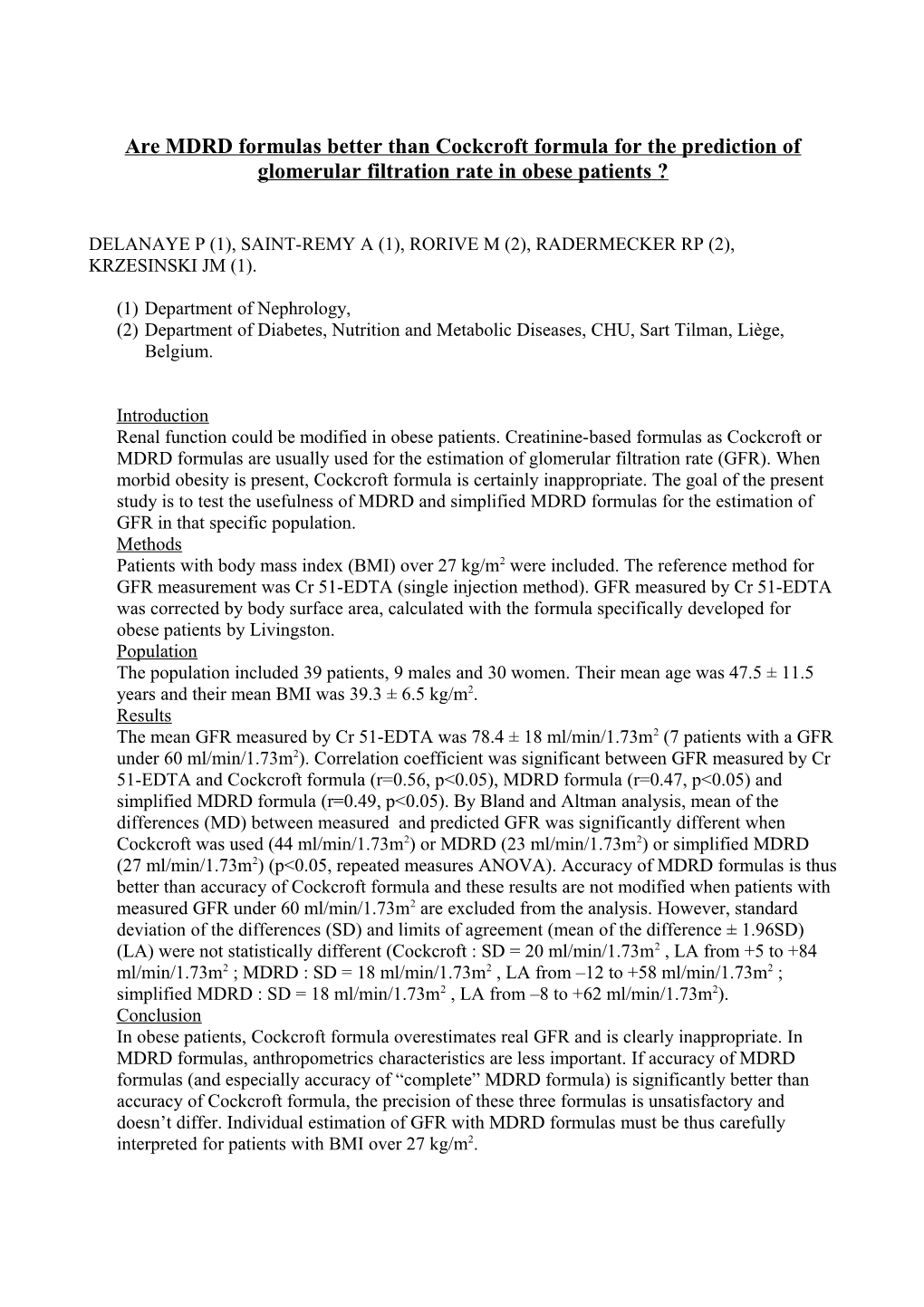# Are MDRD Formulas Better Than Cockcroft Formula for the Prediction of Glomerular FiltrationAre MDRD formulas better than Cockcroft formula for the prediction of glomerular filtration rate in obese patients?

DELANAYE P (1), SAINT-REMY A (1), RORIVE M (2), RADERMECKER RP (2), KRZESINSKI JM (1).

(1)Department of Nephrology,

(2)Department of Diabetes, Nutrition and Metabolic Diseases, CHU, Sart Tilman, Liège, Belgium.

## Introduction

Renal function could be modified in obese patients. Creatinine-based formulas as Cockcroft or MDRD formulas are usually used for the estimation of glomerular filtration rate (GFR). When morbid obesity is present, Cockcroft formula is certainly inappropriate. The goal of the present study is to test the usefulness of MDRD and simplified MDRD formulas for the estimation of GFR in that specific population.

## Methods

Patients with body mass index (BMI) over 27 kg/m2 were included. The reference method for GFR measurement was Cr 51-EDTA (single injection method). GFR measured by Cr 51-EDTA was corrected by body surface area, calculated with the formula specifically developed for obese patients by Livingston.

## Population

The population included 39 patients, 9 males and 30 women. Their mean age was 47.5 ± 11.5 years and their mean BMI was 39.3 ± 6.5 kg/m2.

## Results

The mean GFR measured by Cr 51-EDTA was 78.4 ± 18 ml/min/1.73m2 (7 patients with a GFR under 60 ml/min/1.73m2). Correlation coefficient was significant between GFR measured by Cr 51-EDTA and Cockcroft formula (r=0.56, p<0.05), MDRD formula (r=0.47, p<0.05) and simplified MDRD formula (r=0.49, p<0.05). By Bland and Altman analysis, mean of the differences (MD) between measured and predicted GFR was significantly different when Cockcroft was used (44 ml/min/1.73m2) or MDRD (23 ml/min/1.73m2) or simplified MDRD (27 ml/min/1.73m2) (p<0.05, repeated measures ANOVA). Accuracy of MDRD formulas is thus better than accuracy of Cockcroft formula and these results are not modified when patients with measured GFR under 60 ml/min/1.73m2 are excluded from the analysis. However, standard deviation of the differences (SD) and limits of agreement (mean of the difference ± 1.96SD) (LA) were not statistically different (Cockcroft : SD = 20 ml/min/1.73m2 , LA from +5 to +84 ml/min/1.73m2 ; MDRD : SD = 18 ml/min/1.73m2 , LA from –12 to +58 ml/min/1.73m2 ; simplified MDRD : SD = 18 ml/min/1.73m2 , LA from –8 to +62 ml/min/1.73m2).

## Conclusion

In obese patients, Cockcroft formula overestimates real GFR and is clearly inappropriate. In MDRD formulas, anthropometrics characteristics are less important. If accuracy of MDRD formulas (and especially accuracy of “complete” MDRD formula) is significantly better than accuracy of Cockcroft formula, the precision of these three formulas is unsatisfactory and doesn’t differ. Individual estimation of GFR with MDRD formulas must be thus carefully interpreted for patients with BMI over 27 kg/m2.

Formula / Correlation / Mean of the differences / Standard deviation of the differences / Limits of agreement / Percentage of relative differences over 30% between predicted and measured GFR / Percentage of relative differences over 50% between predicted and measured GFR / Percentage of absolute differences over 10ml/min/1,73m2between predicted and measured GFR / Percentage of absolute differences over 20 ml/min/1,73m2between predicted and measured GFR / Percentage of absolute differences over 30 ml/min/1,73m2between predicted and measured GFR
Cockcroft / 0,56 / 44 / 20 / +5 to +84 / 77 / 62 / 92 / 90 / 72
MDRD / 0,47 / 23 * / 18 / -12 to +58 / 51* / 23* / 77 / 56 / 33
sMDRD / 0,49 / 27 * / 18 / -8 to +62 / 56* / 28* / 79 / 64 / 46* = significantly better than Cockcroft